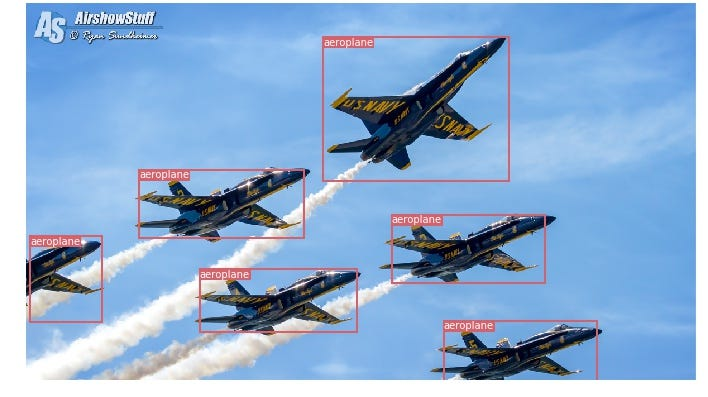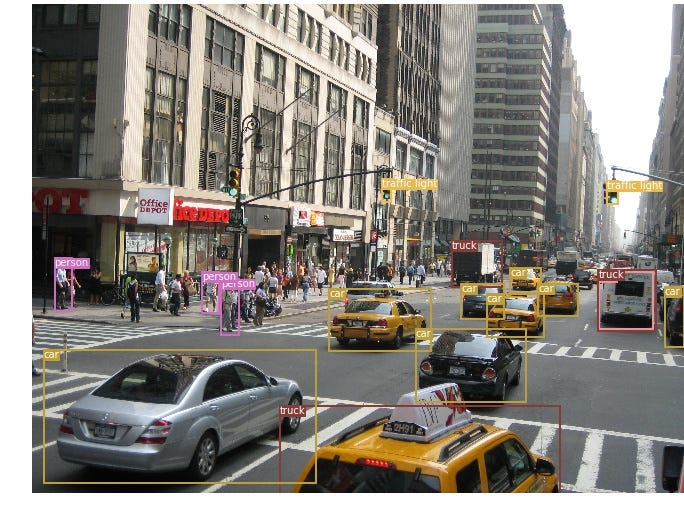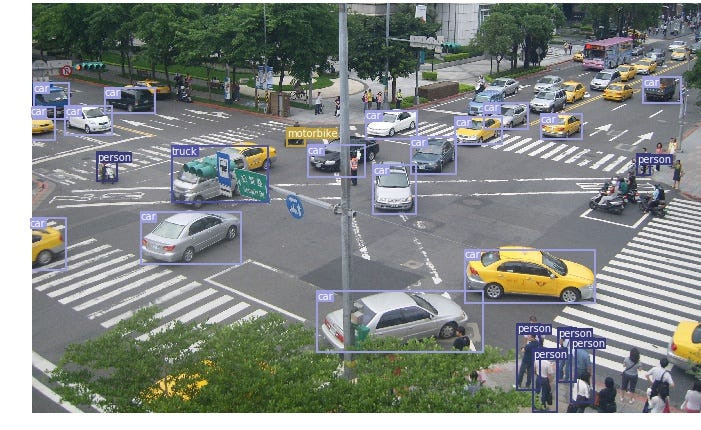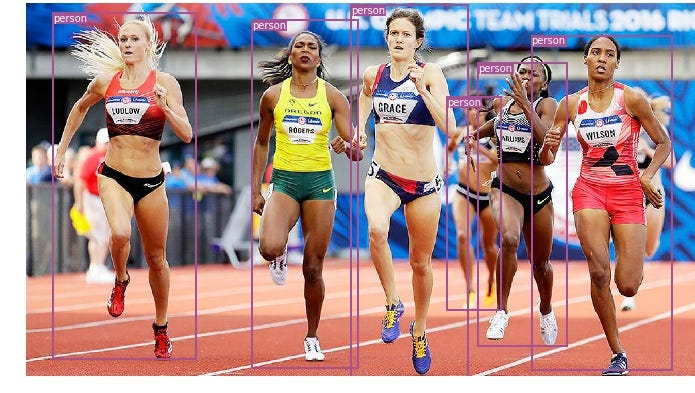## Object detection and tracking in PyTorch| |
[所属分类 开发（python） | 发布者 店小二04 | 时间 2018 | 作者 红领巾 ] 0人收藏点击收藏
Object detection and tracking inPyTorch Detecting multiple objects in images and tracking them invideosChris Fotache

In my previous story, I went over how to train an image classifier in PyTorch , with your own images, and then use it for image recognition. Now I’ll show you how to use a pre-trained classifier to detect multiple objects in an image, and later track them across a video.

What’s the difference between image classification (recognition) and object detection? In classification, you identify what’s the main object in the image and the entire image is classified by a single class. In detection, multiple objects are identified in the image, classified, and a location is also determined (as a bounding box).

Object Detection inImages

There are several algorithms for object detection, with YOLO and SSD among the most popular. For this story, I’ll use YOLOv3 . I won’t get into the technical details of how YOLO (You Only Look Once) works―you can read that here ―but focus instead of how to use it in your own application.

So let’s jump into the code! The Yolo detection code here is based on Erik Lindernoren ’s implementation of Joseph Redmon and Ali Farhadi’s paper . The code snippets below are from a Jupyter Notebook you can find in my Github repo . Before you run this, you’ll need to run the download_weights.sh script in the config folder to download the Yolo weights file. We start by importing the required modules:

from models import * from utils import * import os, sys, time, datetime, random import torch from torch.utils.data import DataLoader from torchvision import datasets, transforms from torch.autograd import Variable import matplotlib.pyplot as plt import matplotlib.patches as patches from PIL import Image

Then we load the pre-trained configuration and weights, as well as the class names of the COCO dataset on which the Darknet model was trained. As always in PyTorch, don’t forget to set the model in eval mode after loading.

config_path='config/yolov3.cfg' weights_path='config/yolov3.weights' class_path='config/coco.names' img_size=416 conf_thres=0.8 nms_thres=0.4 # Load model and weights model = Darknet(config_path, img_size=img_size) model.load_weights(weights_path) model.cuda() model.eval() classes = utils.load_classes(class_path) Tensor = torch.cuda.FloatTensor

There are also a few pre-defined values above: The image size (416px squares), confidence threshold and the non-maximum suppression threshold.

Below is the basic function that will return detections for a specified image. Note that it requires a Pillow image as input. Most of the code deals with resizing the image to a 416px square while maintaining its aspect ratio and padding the overflow. The actual detection is in the last 4 lines.

def detect_image(img): # scale and pad image ratio = min(img_size/img.size, img_size/img.size) imw = round(img.size * ratio) imh = round(img.size * ratio) img_transforms=transforms.Compose([transforms.Resize((imh,imw)), transforms.Pad((max(int((imh-imw)/2),0), max(int((imw-imh)/2),0), max(int((imh-imw)/2),0), max(int((imw-imh)/2),0)), (128,128,128)), transforms.ToTensor(), ]) # convert image to Tensor image_tensor = img_transforms(img).float() image_tensor = image_tensor.unsqueeze_(0) input_img = Variable(image_tensor.type(Tensor)) # run inference on the model and get detections with torch.no_grad(): detections = model(input_img) detections = utils.non_max_suppression(detections, 80, conf_thres, nms_thres) return detections

Finally, let’s put it together by loading an image, getting the detections, and then displaying it with the bounding boxes around detected objects. Again, most of the code here deals with scaling and padding the image, as well as getting different colors for each detected class.

You can put together these code fragments to run the code, or download the notebook from my Github. Here are a few examples of object detection in images:Object Tr

1.凡CodeSecTeam转载的文章,均出自其它媒体或其他官网介绍,目的在于传递更多的信息,并不代表本站赞同其观点和其真实性负责；
2.转载的文章仅代表原创作者观点,与本站无关。其原创性以及文中陈述文字和内容未经本站证实,本站对该文以及其中全部或者部分内容、文字的真实性、完整性、及时性，不作出任何保证或承若；
3.如本站转载稿涉及版权等问题,请作者及时联系本站,我们会及时处理。技术大类 | 开发（python） | 评论(0) | 阅读(36)